## Monday, 15 October 2012

### Counting in combinatorics I - Review in basic arithmetics

Counting is the action of finding the number of elements of a finite set of objects.

Counting is perhaps described as a pre-math skill, but is consistantly used in numerous higher fields, like linear algebra, abstract algebra, and especially combinatorics --- fitting the definition above, combinatorics emphasizes the sample spaces and targetted set. In other words, number of combinations is simply the number of elements in the targeted set.

I. the basic implication of counting

Back to what you've learnt in the kindergarden, we are turning a "situation" into a specific number, which is an elemental quantification process. If '+' is representing 'an' apple then we count '+++' --- 'an apple and an apple and an apple' --- to 3.

Sometimes we will use the concept of steps to conduct the counting --- we start from '+' which is 1, two '+'s left means 2 steps forward which is 2, and 3. The 'step approach' develops as the principle of addition in such given situations.

What can be called a suprise is that what we've learnt in kindergarden has a high coincidence with vigoruously defined arithemetic system, which relies on the Peano's arithmeitc axioms.

Another approach for counting is by defining specific finite numbers. Instead of saying '+++' is 'one more than one plus one' we 'define' it to be 'three' --- this is particular effective for finite numbers, as well as for pre-education as they don't have the concept of addition.

In a formal way to describe the above approach, we say the 'situations' (number of '+', apples, whatever) is mapped to a sequence {1,2,3,4,.....}, which is the natrual numbers.

Mapping between different spaces is one of the most important idea in mathematics: geometry (inversion), linear algebra (linear transformation), algebra (functions), statistics (distribution transformation), etc. And we use mapping again in counting.

More precisely, combinatorics, the extension of counting.

II. Principle of counting

This time I didn't aim to introduce so advanced tricks to compute hard combinatorical problem, but I'm trying to provide alternatiive views for counting-based college combinatorics problems.

How do we count?

With finite and 'small' set of elements we can count it by 'definition' of specific numbers like above, but for harder problems we need an aloairthm for it.

Denote the count of a set A be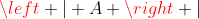. (If you know some set theory this shouldn't be something new to you.)

The two basic principle:

Principle of addition (in combinatorics):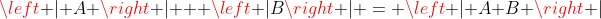.

Why? We start to count from A, starting from zero: 0, 1, 2, .... up to |A|. We can also count B, starting from zero again: 0, 1, 2,... up to |B|. Now, if we start to count B after A --- starting from |A|, we have |A|, |A|+1, |A|+2,... up to |A| + |B| which is our answer.

Formally, as elements repetition will be counted with its multiplicity, we assume all elements are distinct, then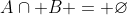which leads to the above result.

I have to point out that the concept of zero appears quite frequently which is different from the natrual number system elementally ruled by the peano's axioms. This is an interesting problem to think with: As natrual numbers are used since the pre-historic era but the concept of zero appears very lately (later than negative numbers and fractions), why the concept of zero is so elemental in modern society? One of the reason is probably about the number bases creates a 'nothing' in a certain digit but the overall number represents something --- in ancient times they uses a blank to represent 'nothing' for that digit but that looks so odd and the concept of zero eventually appears.

Definition. We can 'multiply' sets (formally called cartesian product): If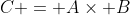where A, B are sets, then C is also a set which includes: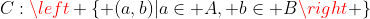Example. Denote the result of the first toss of a coin be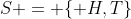and that for the second coin be the same. The result of tossing two coins is given by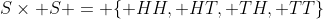.

In basic arithmetic, multiplication is only a 'repetition' or 'short form' of addition (similarly, power or factorial is only a 'repetition' or 'short form' of multiplication.

Here's quite a famous quote from master rigorist, Landau's Foundation of Analysis:

'The preface for student...

4. The multiplication table will not occur in this book, not even the following theorem:
2*2 = 4
but I would recommend, as an exercise, define:
2 = 1+1
4 = ((1+1)+1)+1
then prove the theorem as an exercise...

The preface for scholar...

...I hope that I've written this book in such a way that a normal student can read it in two days. And then (since he already knows the formal rules from school) he may forget its contents."

(Interested reader should try to prove the above statement.)

Multiplication is not a necessary in arithmetic because there is addition. Similarly we transform the expression of multiplication into the basic way (addition) and our counting would work.

Principle of multiplication (in combinatorics):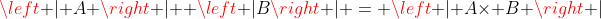.

What does it imply? If A and B are sets with some elements, and C is the set of choice to choose one from A and one from B, then the resulting count is given by |A x B|.

Proof: left as exerise --- if you have no idea try to prove the theorem that Landau stated.

Up to now it seems that we are simply repeating what we're taught in schools, but starting from the basic, magic will happen soon.